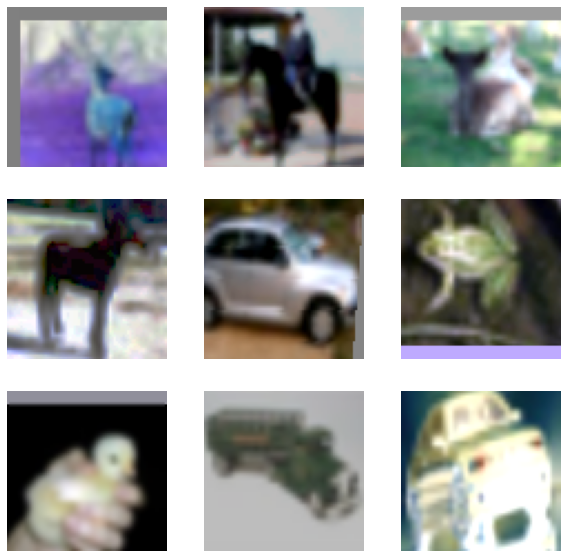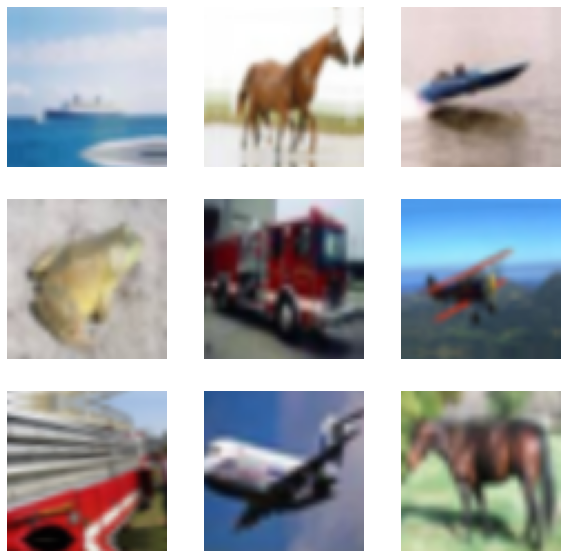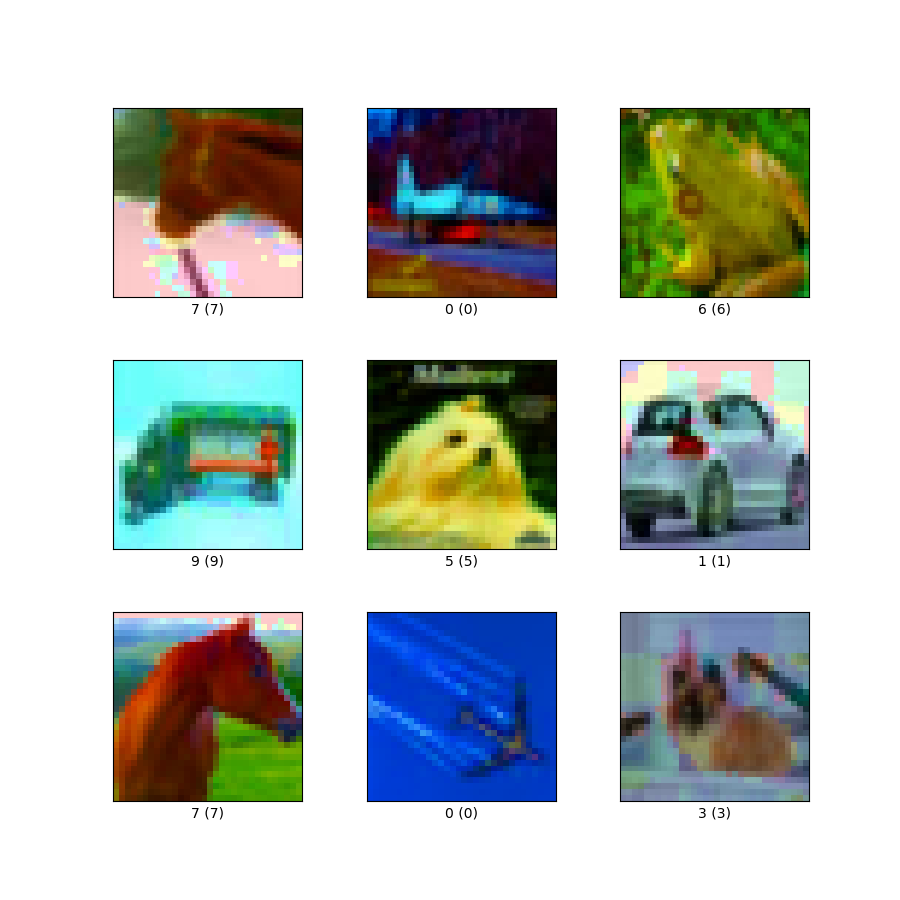Code examples / Computer Vision / RandAugment for Image Classification for Improved Robustness

# RandAugment for Image Classification for Improved Robustness

Author: Sayak Paul
Date created: 2021/03/13
Description: RandAugment for training an image classification model with improved robustness.

ⓘ This example uses Keras 2View in ColabGitHub source

Data augmentation is a very useful technique that can help to improve the translational invariance of convolutional neural networks (CNN). RandAugment is a stochastic data augmentation routine for vision data and was proposed in RandAugment: Practical automated data augmentation with a reduced search space. It is composed of strong augmentation transforms like color jitters, Gaussian blurs, saturations, etc. along with more traditional augmentation transforms such as random crops.

RandAugment has two parameters:

• `n` that denotes the number of randomly selected augmentation transforms to apply sequentially
• `m` strength of all the augmentation transforms

These parameters are tuned for a given dataset and a network architecture. The authors of RandAugment also provide pseudocode of RandAugment in the original paper (Figure 2).

Recently, it has been a key component of works like Noisy Student Training and Unsupervised Data Augmentation for Consistency Training. It has been also central to the success of EfficientNets.

This example requires TensorFlow 2.4 or higher, as well as `imgaug`, which can be installed using the following command:

``````pip install imgaug
``````

## Imports & setup

``````import tensorflow as tf
import numpy as np
import matplotlib.pyplot as plt
from tensorflow.keras import layers
import tensorflow_datasets as tfds
from imgaug import augmenters as iaa
import imgaug as ia

tfds.disable_progress_bar()
tf.random.set_seed(42)
ia.seed(42)
``````

For this example, we will be using the CIFAR10 dataset.

``````(x_train, y_train), (x_test, y_test) = tf.keras.datasets.cifar10.load_data()
print(f"Total training examples: {len(x_train)}")
print(f"Total test examples: {len(x_test)}")
``````
``````Total training examples: 50000
Total test examples: 10000
``````

## Define hyperparameters

``````AUTO = tf.data.AUTOTUNE
BATCH_SIZE = 128
EPOCHS = 1
IMAGE_SIZE = 72
``````

## Initialize `RandAugment` object

Now, we will initialize a `RandAugment` object from the `imgaug.augmenters` module with the parameters suggested by the RandAugment authors.

``````rand_aug = iaa.RandAugment(n=3, m=7)

def augment(images):
# Input to `augment()` is a TensorFlow tensor which
# is not supported by `imgaug`. This is why we first
# convert it to its `numpy` variant.
images = tf.cast(images, tf.uint8)
return rand_aug(images=images.numpy())
``````

## Create TensorFlow `Dataset` objects

Because `RandAugment` can only process NumPy arrays, it cannot be applied directly as part of the `Dataset` object (which expects TensorFlow tensors). To make `RandAugment` part of the dataset, we need to wrap it in a [`tf.py_function`](https://www.tensorflow.org/api_docs/python/tf/py_function).

A `tf.py_function` is a TensorFlow operation (which, like any other TensorFlow operation, takes TF tensors as arguments and returns TensorFlow tensors) that is capable of running arbitrary Python code. Naturally, this Python code can only be executed on CPU (whereas the rest of the TensorFlow graph can be accelerated on GPU), which in some cases can cause significant slowdowns — however, in this case, the `Dataset` pipeline will run asynchronously together with the model, and doing preprocessing on CPU will remain performant.

``````train_ds_rand = (
tf.data.Dataset.from_tensor_slices((x_train, y_train))
.shuffle(BATCH_SIZE * 100)
.batch(BATCH_SIZE)
.map(
lambda x, y: (tf.image.resize(x, (IMAGE_SIZE, IMAGE_SIZE)), y),
num_parallel_calls=AUTO,
)
.map(
lambda x, y: (tf.py_function(augment, [x], [tf.float32]), y),
num_parallel_calls=AUTO,
)
.prefetch(AUTO)
)

test_ds = (
tf.data.Dataset.from_tensor_slices((x_test, y_test))
.batch(BATCH_SIZE)
.map(
lambda x, y: (tf.image.resize(x, (IMAGE_SIZE, IMAGE_SIZE)), y),
num_parallel_calls=AUTO,
)
.prefetch(AUTO)
)
``````

Note about using `tf.py_function`:

• As our `augment()` function is not a native TensorFlow operation chances are likely that it can turn into an expensive operation. This is why it is much better to apply it after batching our dataset.
• `tf.py_function` is not compatible with TPUs. So, if you have distributed TensorFlow training pipelines that use TPUs you cannot use `tf.py_function`. In that case, consider switching to a multi-GPU environment, or rewriting the contents of the function in pure TensorFlow.

For comparison purposes, let's also define a simple augmentation pipeline consisting of random flips, random rotations, and random zoomings.

``````simple_aug = tf.keras.Sequential(
[
layers.Resizing(IMAGE_SIZE, IMAGE_SIZE),
layers.RandomFlip("horizontal"),
layers.RandomRotation(factor=0.02),
layers.RandomZoom(
height_factor=0.2, width_factor=0.2
),
]
)

# Now, map the augmentation pipeline to our training dataset
train_ds_simple = (
tf.data.Dataset.from_tensor_slices((x_train, y_train))
.shuffle(BATCH_SIZE * 100)
.batch(BATCH_SIZE)
.map(lambda x, y: (simple_aug(x), y), num_parallel_calls=AUTO)
.prefetch(AUTO)
)
``````

## Visualize the dataset augmented with RandAugment

``````sample_images, _ = next(iter(train_ds_rand))
plt.figure(figsize=(10, 10))
for i, image in enumerate(sample_images[:9]):
ax = plt.subplot(3, 3, i + 1)
plt.imshow(image.numpy().astype("int"))
plt.axis("off")
``````You are encouraged to run the above code block a couple of times to see different variations.

## Visualize the dataset augmented with `simple_aug`

``````sample_images, _ = next(iter(train_ds_simple))
plt.figure(figsize=(10, 10))
for i, image in enumerate(sample_images[:9]):
ax = plt.subplot(3, 3, i + 1)
plt.imshow(image.numpy().astype("int"))
plt.axis("off")
``````## Define a model building utility function

Now, we define a CNN model that is based on the ResNet50V2 architecture. Also, notice that the network already has a rescaling layer inside it. This eliminates the need to do any separate preprocessing on our dataset and is specifically very useful for deployment purposes.

``````def get_training_model():
resnet50_v2 = tf.keras.applications.ResNet50V2(
weights=None,
include_top=True,
input_shape=(IMAGE_SIZE, IMAGE_SIZE, 3),
classes=10,
)
model = tf.keras.Sequential(
[
layers.Input((IMAGE_SIZE, IMAGE_SIZE, 3)),
layers.Rescaling(scale=1.0 / 127.5, offset=-1),
resnet50_v2,
]
)
return model

print(get_training_model().summary()
``````
``````Model: "sequential_1"
_________________________________________________________________
Layer (type)                 Output Shape              Param #
=================================================================
rescaling (Rescaling)        (None, 72, 72, 3)         0
_________________________________________________________________
resnet50v2 (Functional)      (None, 10)                23585290
=================================================================
Total params: 23,585,290
Trainable params: 23,539,850
Non-trainable params: 45,440
_________________________________________________________________
``````

We will train this network on two different versions of our dataset:

• One augmented with RandAugment.
• Another one augmented with `simple_aug`.

Since RandAugment is known to enhance the robustness of models to common perturbations and corruptions, we will also evaluate our models on the CIFAR-10-C dataset, proposed in Benchmarking Neural Network Robustness to Common Corruptions and Perturbations by Hendrycks et al. The CIFAR-10-C dataset consists of 19 different image corruptions and perturbations (for example speckle noise, fog, Gaussian blur, etc.) that too at varying severity levels. For this example we will be using the following configuration: `cifar10_corrupted/saturate_5`. The images from this configuration look like so:In the interest of reproducibility, we serialize the initial random weights of our shallow network.

``````initial_model = get_training_model()
initial_model.save_weights("initial_weights.h5")
``````

## Train model with RandAugment

``````rand_aug_model = get_training_model()
rand_aug_model.compile(
)
rand_aug_model.fit(train_ds_rand, validation_data=test_ds, epochs=EPOCHS)
_, test_acc = rand_aug_model.evaluate(test_ds)
print("Test accuracy: {:.2f}%".format(test_acc * 100))
``````
``````391/391 [==============================] - 1199s 3s/step - loss: 2.0652 - accuracy: 0.2744 - val_loss: 1.6281 - val_accuracy: 0.4319
79/79 [==============================] - 46s 580ms/step - loss: 1.6281 - accuracy: 0.4319
Test accuracy: 43.19%
``````

## Train model with `simple_aug`

``````simple_aug_model = get_training_model()
simple_aug_model.compile(
)
simple_aug_model.fit(train_ds_simple, validation_data=test_ds, epochs=EPOCHS)
_, test_acc = simple_aug_model.evaluate(test_ds)
print("Test accuracy: {:.2f}%".format(test_acc * 100))
``````
``````391/391 [==============================] - 1169s 3s/step - loss: 1.7628 - accuracy: 0.3862 - val_loss: 1.3458 - val_accuracy: 0.5305
79/79 [==============================] - 42s 527ms/step - loss: 1.3458 - accuracy: 0.5305
Test accuracy: 53.05%
``````

## Load the CIFAR-10-C dataset and evaluate performance

``````# Load and prepare the CIFAR-10-C dataset
cifar_10_c = cifar_10_c.batch(BATCH_SIZE).map(
lambda x, y: (tf.image.resize(x, (IMAGE_SIZE, IMAGE_SIZE)), y),
num_parallel_calls=AUTO,
)

# Evaluate `rand_aug_model`
_, test_acc = rand_aug_model.evaluate(cifar_10_c, verbose=0)
print(
"Accuracy with RandAugment on CIFAR-10-C (saturate_5): {:.2f}%".format(
test_acc * 100
)
)

# Evaluate `simple_aug_model`
_, test_acc = simple_aug_model.evaluate(cifar_10_c, verbose=0)
print(
"Accuracy with simple_aug on CIFAR-10-C (saturate_5): {:.2f}%".format(
test_acc * 100
)
)
``````
``````Accuracy with RandAugment on CIFAR-10-C (saturate_5): 35.90%
Accuracy with simple_aug on CIFAR-10-C (saturate_5): 47.34%
``````

For the purpose of this example, we trained the models for only a single epoch. On the CIFAR-10-C dataset, the model with RandAugment can perform better with a higher accuracy (for example, 76.64% in one experiment) compared with the model trained with `simple_aug` (e.g., 64.80%). RandAugment can also help stabilize the training. You can explore this notebook to check some of the results.

In the notebook, you may notice that, at the expense of increased training time with RandAugment, we are able to carve out far better performance on the CIFAR-10-C dataset. You can experiment on the other corruption and perturbation settings that come with the run the same CIFAR-10-C dataset and see if RandAugment helps.

You can also experiment with the different values of `n` and `m` in the `RandAugment` object. In the original paper, the authors show the impact of the individual augmentation transforms for a particular task and a range of ablation studies. You are welcome to check them out.

RandAugment has shown great progress in improving the robustness of deep models for computer vision as shown in works like Noisy Student Training and FixMatch. This makes RandAugment quite a useful recipe for training different vision models.

You can use the trained model hosted on Hugging Face Hub and try the demo on Hugging Face Spaces.